• 并行梯度优化的佳作
• 通俗易懂

# 简介

• 基于值与基于策略的方法
• on-policy与off-policy的方法
• 离散与连续问题

• 稳定训练
• 在Atari游戏上使用DQN算法，一个16核CPU比Nvidia K40 GPU快，使用A3C算法可以快一倍
• 成功适用于很多连续运动学控制问题，例如图像输入的3D迷宫

the sequence of observed data encountered by an online RL agent is non-stationary, and on-line RL updates are strongly correlated. By storing the agent’s data in an experience replay memory, the data can be batched or randomly sampled from different time-steps. Aggregating over memory in this way reduces non-stationarity and decorrelates updates, but at the same time limits the methods to off-policy reinforcement learning algorithms

1. 占用内存，增加计算量
2. 需要off-policy算法

1. 实现减轻数据相关性的效果，使训练稳定
2. 可用于大范围on-policy算法，如Sarsa，n-step方法，A-C方法，也可用于off-policy方法，如Q-learning
3. 利用深度神经网络设计算法，保持鲁棒性与有效性（不予置评）
4. 不使用GPU，只使用多核CPU，反而训练时间短
5. 比大规模分布式需要更少的资源占用（内存、算力等）
6. 相比于Gorila异步训练方式，本文中的训练方式不需要中心服务器，其使用的是共享内存模式
7. 单机运行减少了通信（梯度和超参数）的开销

# 文中精要

Hogwild! 这个方法的提出也很偶然，容我步步道来：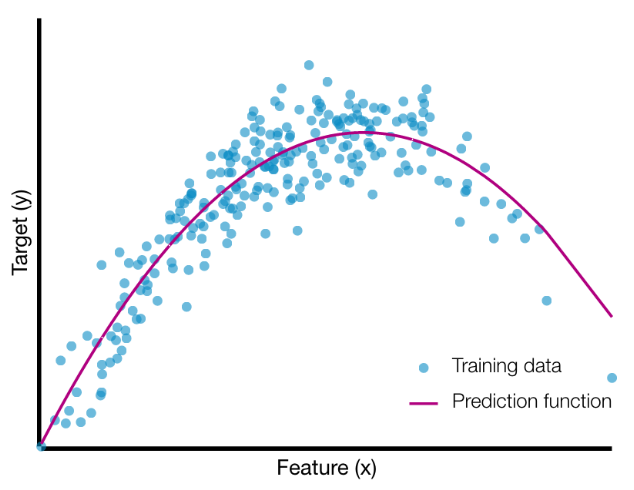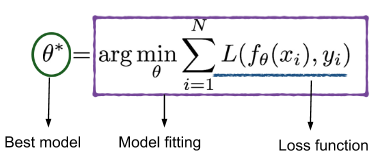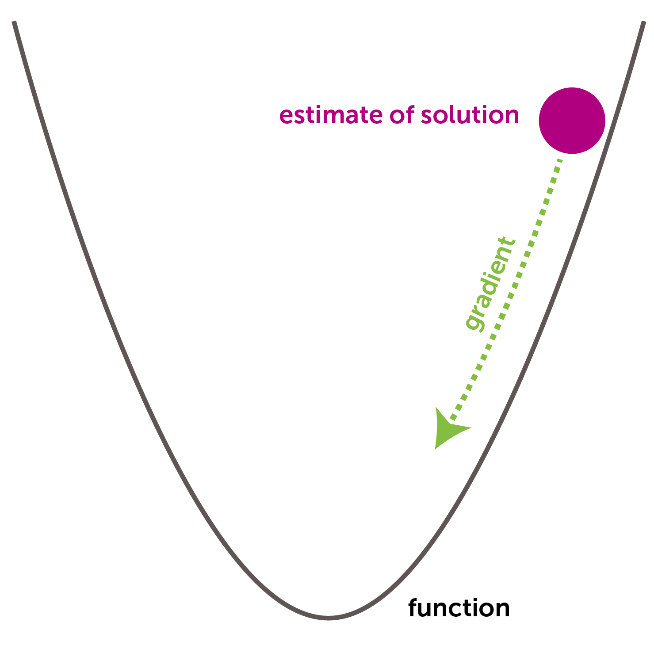SGD的算法流程如下：

• 选一个初始参数向量$\theta$和正步长$\alpha$
• 循环直到满足结束条件：
• 从训练集中随机选择一个样本$x_{i}$
• 更新参数$\theta \leftarrow\left(\theta-\alpha \nabla L\left(f_{\theta}\left(x_{i}\right), y_{i}\right)\right)$

SGD的优点：

1. 少内存占用。SGD不需要所有的样本集进行计算梯度，只需要从样本集中抽取一个样本进行训练。频繁的采样操作可以使用高速缓存来加速训练。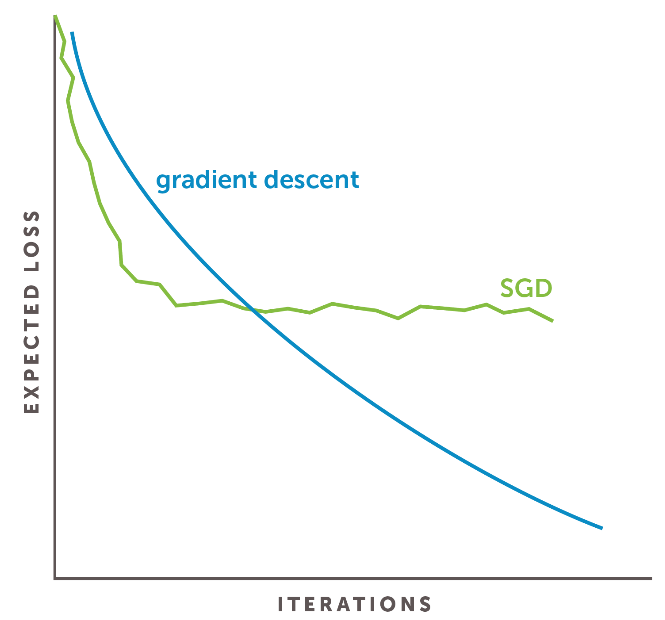• 每个线程从训练集随机抽取一个样本$x_{i}$
• 锁参数$\theta$
• 线程读参数$\theta$
• 线程更新参数$\theta \leftarrow\left(\theta-\alpha \nabla L\left(f_{\theta}\left(x_{i}\right), y_{i}\right)\right)$
• 解锁

• 每个线程从训练集随机抽取一个样本$x_{i}$
• 锁参数$\theta$
• 线程读参数$\theta$
• 线程更新参数$\theta \leftarrow\left(\theta-\alpha \nabla L\left(f_{\theta}\left(x_{i}\right), y_{i}\right)\right)$
• 解锁

In a sentence, the main idea of Hogwild! is — “Remove all thread locks from parallel SGD code.” In Hogwild!, threads can overwrite each other by writing at the same time and compute gradients using a stale version of the “current solution.”

## 伪代码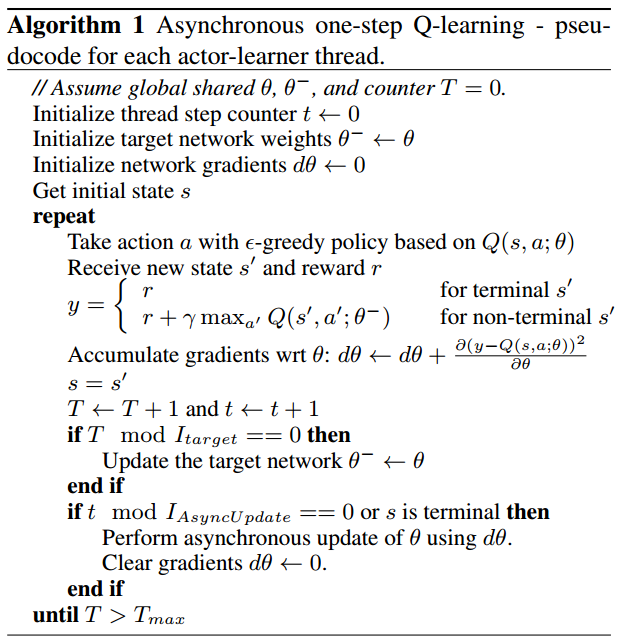• 相比后两个伪代码，该代码中各线程是不需要复制用于选择动作的训练网络（因为各方对target network的定义不同，有些人认为等待赋值的是目标网络，有些人认为需要训练的是目标网络，因此，此处不使用目标网络的术语）的，即每次选择动作，都使用其他线程可能更新过的Q网络进行决策。这是因为不需要使用同一个决策模型向后看多步
• $\theta$是动态变化的，即在该线程的训练过程中，其他线程也可能对参数$\theta$进行了更新
• $I_{target}$代表双Q学习赋值的间隔
• $I_{AsyncUpdate}$代表单线程对共享参数$\theta$更新的间隔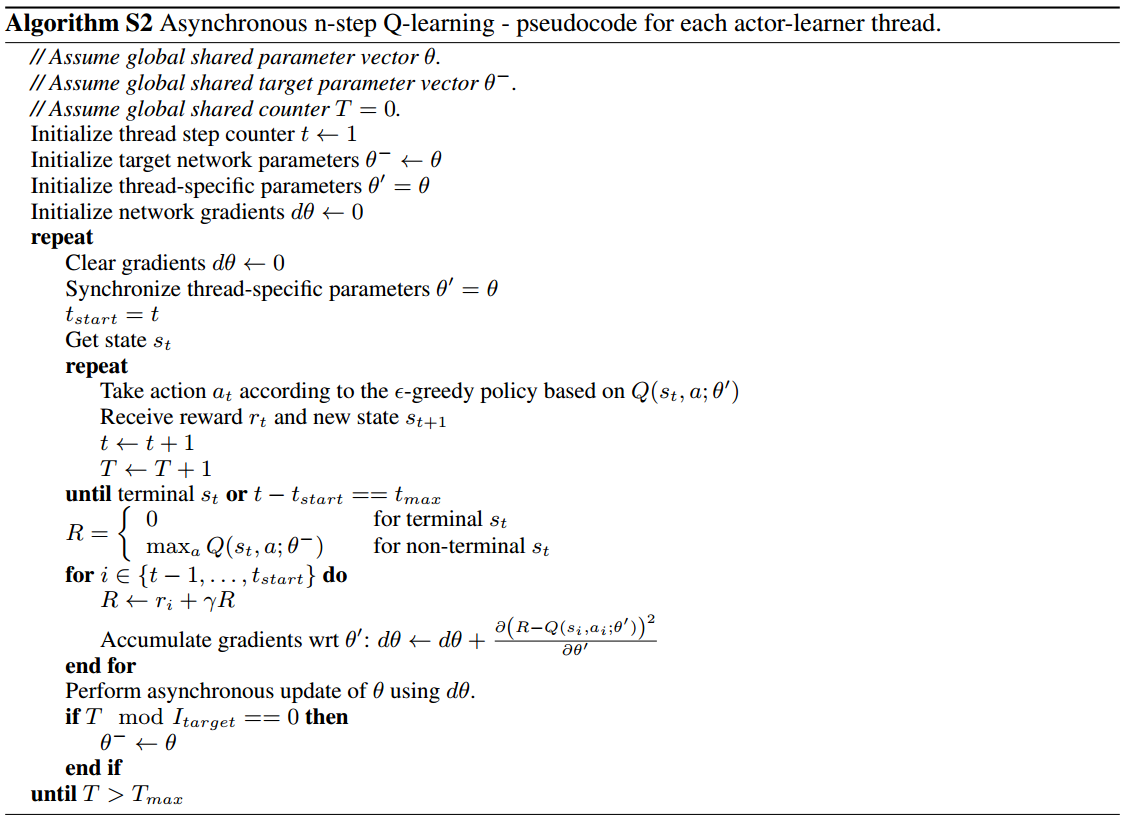• 相比于one-step，该算法为每个线程配置了一个备份网络$\color{red}{\theta’}$
• $t_{max}$为n-step中的$n$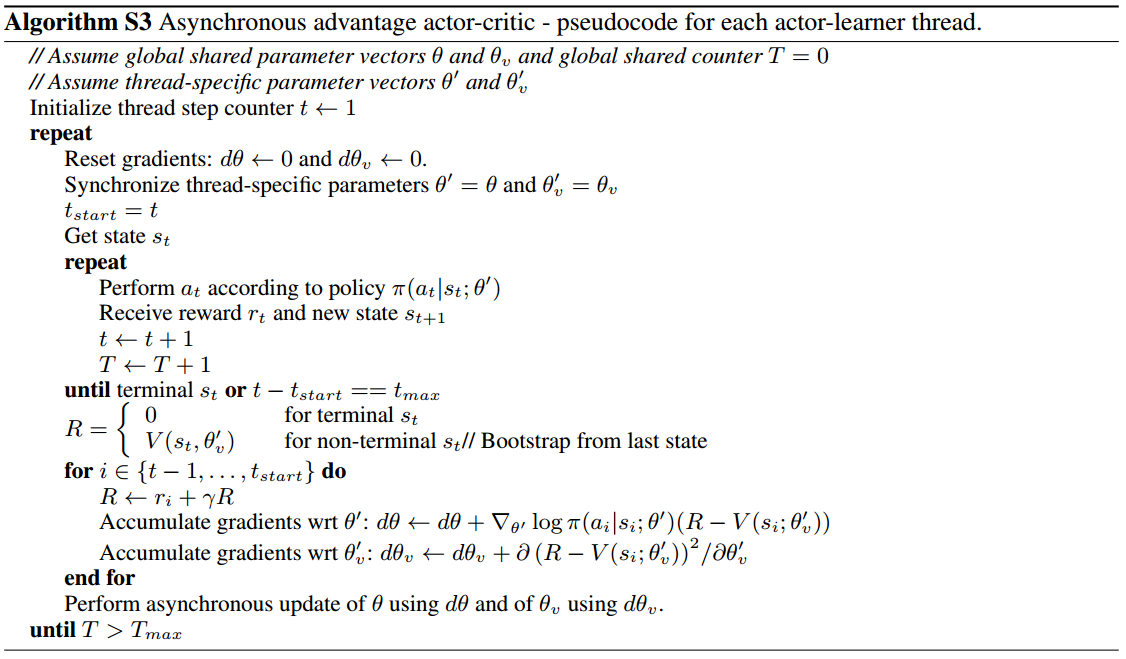• $t_{max}$为n-step向前看的步数
• 文中针对该模型将A-C网络架构共享了部分神经网络参数，并且对actor网络的损失函数公式进行了改造：$\nabla_{\theta^{\prime}} \log \pi\left(a_{t} | s_{t} ; \theta^{\prime}\right)\left(R_{t}-V\left(s_{t} ; \theta_{v}\right)\right)+\color{red}{\beta \nabla_{\theta^{\prime}} H\left(\pi\left(s_{t} ; \theta^{\prime}\right)\right)}$，即添加了熵正则化项，它的作用是增加探索，避免网络过早地收敛至局部最优，$\beta$为超参数

# 实验部分

Atari 2600 实验结果：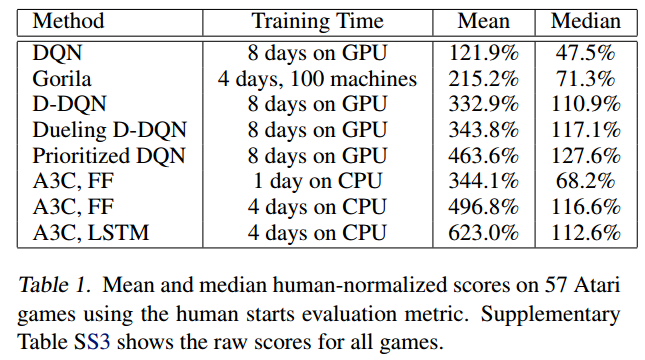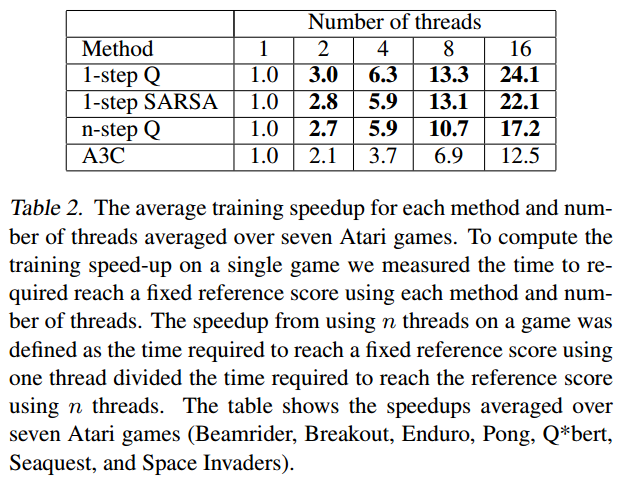• 动量SGD，Momentum SGD
• RMSProp
• Shared RMSProp

RMSProp是这样更新的：

$\eta$为学习率，$\alpha$为RMSProp折扣因子

RMSProp与Shared RMSProp的差别就是：

• Shared RMSProp各线程共享参数$g$，且无锁异步更新
• RMSProp各线程独立一个参数$g$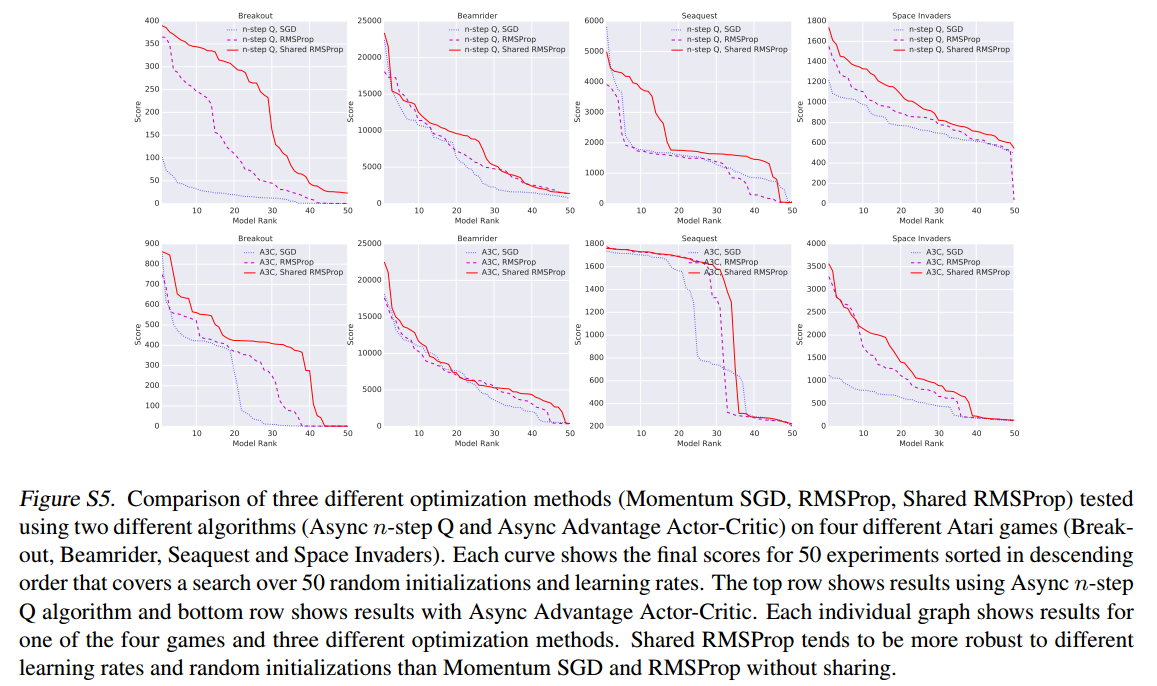• 使用离线在线策略，基于值基于策略方法，离散连续问题
• 稳定训练
• 加速训练
• 比经验池少消耗资源

# 引用

Parallel Machine Learning with Hogwild!

-------------本文结束感谢您的阅读-------------

0%